## Completing the Square: Algebra

Want to learn the ins and out of completing the square?  Then you’ve come to the right place! Learn how to Complete the Square step by step in the video and article below, then try the practice problems at the end of this post to truly master the topic! If you’re looking for more on completing the square, check out this post here. Happy Calculating! 🙂

Check out the video below for an in-depth look at completing the square: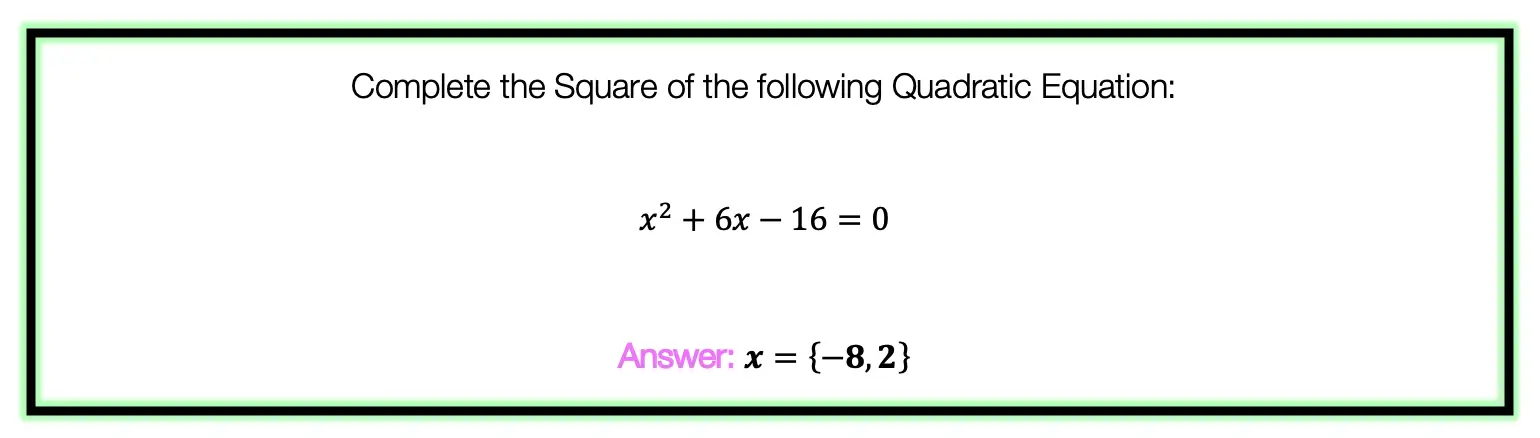To answer this question, there are several steps we must follow including:

Step 1: Move the whole number, which in this case is 16, to the other side of the equation.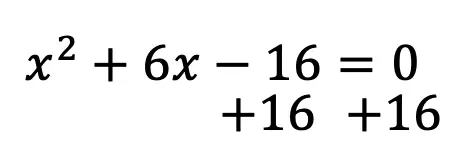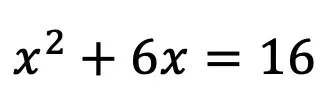Step 2: Make space for our new number on both sides of the equation.  This number is going to be found by using a particular formula shown below: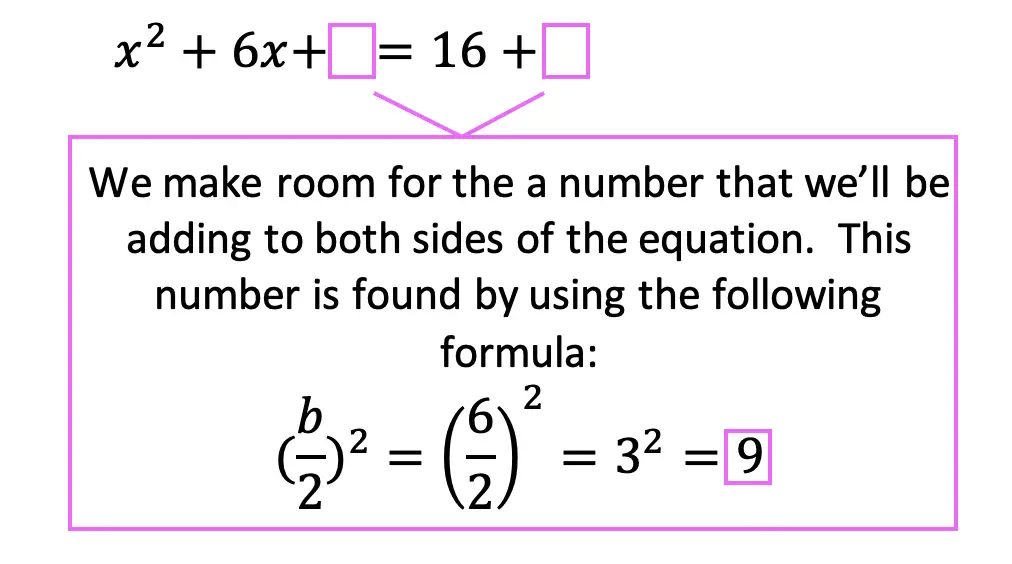Step 3: Add the number 9 to both sides of the equation, which we found using our formula.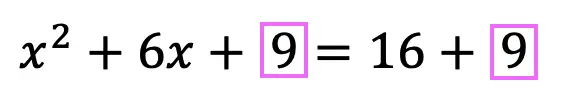Step 4: Combine like terms on the right side of the equation, adding 16+9 to get 25.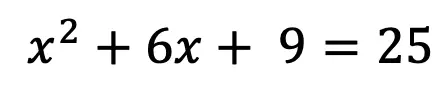Step 5: Now, we need to re-write the left side of the equation using the following formula.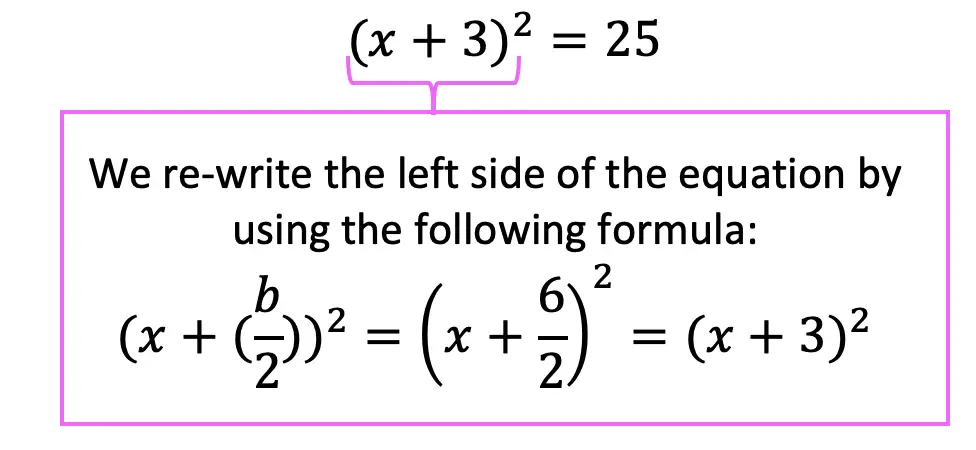Step 6: Finally, we solve for x by taking the positive and negative square root to get the following answer and solve for two different equations: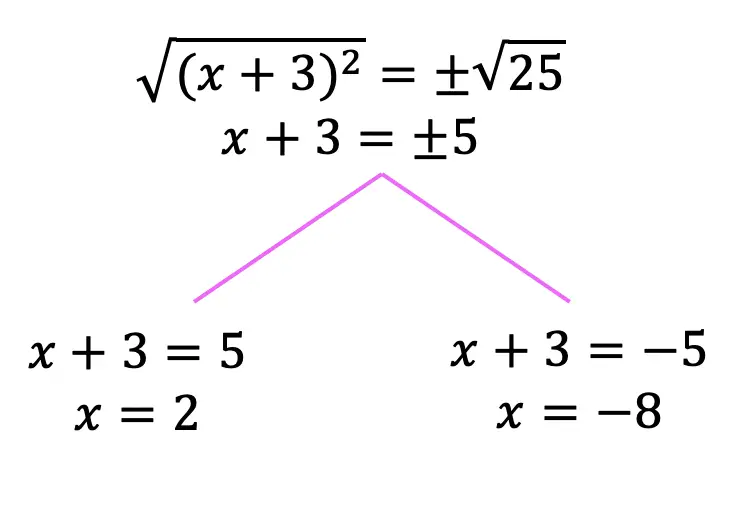Practice Questions: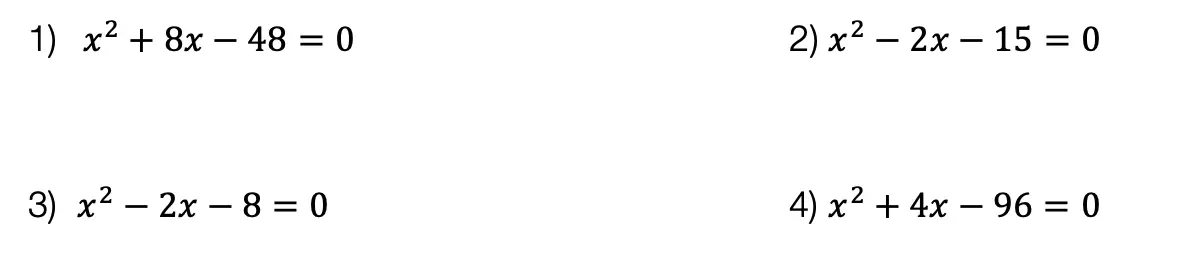Solutions: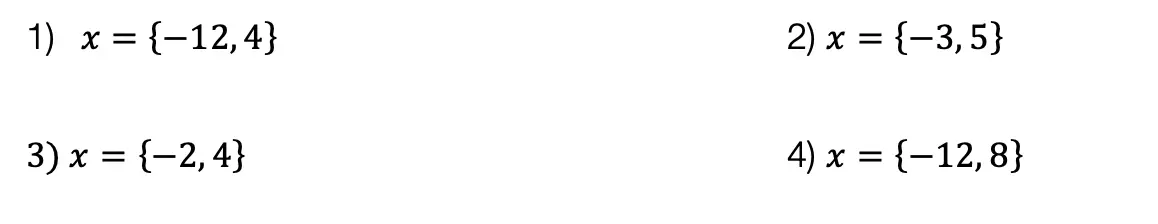Want more Mathsux?  Don’t forget to check out our Youtube channel and more below! And if you have any questions, please don’t hesitate to comment below. Happy Calculating! 🙂

Need more of an explanation?  Check out why we complete the square in the first place here ! 🙂

Looking for more on Quadratic Equations and Functions? Check out the following Related posts!

Factoring Review

Factor by Grouping

Is it a Function?

The Discriminant

4 Ways to Factor Trinomials

Imaginary and Complex Numbers

Quadratic Equations with 2 Imaginary Solutions

Focus and Directrix of a Parabola